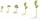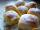# Addition of mixed numerals

Add two mixed fractions:

2 4/6 + 1 3/6

Result

f =  4.167

#### Solution:Leave us a comment of example and its solution (i.e. if it is still somewhat unclear...):

Showing 0 comments:Be the first to comment!#### To solve this example are needed these knowledge from mathematics:

Need help calculate sum, simplify or multiply fractions? Try our fraction calculator.

## Next similar examples:

1. Addition of Roman numbersAdded together and write as decimal number: LXVII + MLXIV
2. Adding mixed numbersAdd this two mixed numbers: 1 5/6 + 2 2/11=
3. Mixed2improperWrite the mixed number as an improper fraction. 166 2/3
4. Roman numerals +Add up the number writtens in Roman numerals. Write the results as a decimal number.
5. Roman numerals 2+Add up the number writtens in Roman numerals. Write the results as a roman numbers.
6. Roses and tulipsAt the florist are 50 tulips and 5 times less roses. How many flowers are in flower shop?
7. Sum of fractionsWhat is the sum of 2/3+3/5?
8. NotebooksLiza a store owner buys 560 notebooks. He sold 3/8 of the notebook then she adds the stocks of notebook of 1/4 of the number of notebooks she bought. What is the total number of notebook she bought?
9. SeedsOf the 72 wet seeds 7/8 germinated. How many seeds germinate?
10. ChocolateChildren break chocolate first to third and then every part of another half. What kind got each child? Draw a picture. What part would have received if each piece have halved?
11. Fraction and a decimalWrite as a fraction and a decimal. One and two plus three and five hundredths
12. In fractionsAn ant climbs 2/5 of the pole on the first hour and climbs 1/4 of the pole on the next hour. What part of the pole does the ant climb in two hours?
13. Lengths of the poolMiguel swam 6 lengths of the pool. Mat swam 3 times as far as Miguel. Lionel swam 1/3 as far as Miguel. How many lengths did mat swim?
14. ZdeněkZdeněk picked up 15 l of water from a 100-liter full-water barrel. Write a fraction of what part of Zdeněk's water he picked.
15. CakesOn the bowl were a few cakes. Jane ate one-third of them, Dana ate a quarter of those cakes that remained. a) What part (of the original number of cakes) Dana ate? b) At least how many cakes could be (initially) on thebowl?
16. Fraction to decimalWrite the fraction 3/22 as a decimal.
17. Jam cakesMom baked a third of plum jam cakes, one third cheesecakes and 18 poppy. How many cakes she had bake?